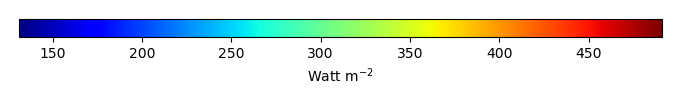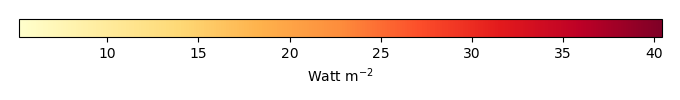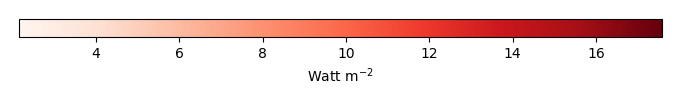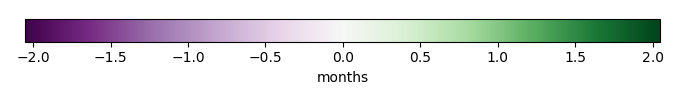# Mean State

Period Mean (original grids) [Watt m-2]
Model Period Mean (intersection) [Watt m-2]
Model Period Mean (complement) [Watt m-2]
Benchmark Period Mean (intersection) [Watt m-2]
Benchmark Period Mean (complement) [Watt m-2]
Bias [Watt m-2]
RMSE [Watt m-2]
Phase Shift [months]
Bias Score 
RMSE Score 
Seasonal Cycle Score 
Spatial Distribution Score 
Interannual Variability Score 
Overall Score 
Benchmark [-] 453.
CLM5PHSOFF [-] 462. 462. 453. 460. 9.50 15.9 1.55 0.359 0.368 0.776 0.993 0.730 0.599
CLM5PHSON [-] 462. 462. 453. 460. 9.34 15.7 1.72 0.344 0.372 0.753 0.988 0.715 0.591
Period Mean (original grids) [Watt m-2]
Model Period Mean (intersection) [Watt m-2]
Model Period Mean (complement) [Watt m-2]
Benchmark Period Mean (intersection) [Watt m-2]
Benchmark Period Mean (complement) [Watt m-2]
Bias [Watt m-2]
RMSE [Watt m-2]
Phase Shift [months]
Bias Score 
RMSE Score 
Seasonal Cycle Score 
Spatial Distribution Score 
Interannual Variability Score 
Overall Score 
Benchmark [-] 471.
CLM5PHSOFF [-] 482. 482. 471. 456. 10.5 20.6 0.623 0.556 0.523 0.954 0.913 0.738 0.701
CLM5PHSON [-] 481. 482. 471. 456. 10.1 20.9 0.713 0.562 0.498 0.945 0.949 0.707 0.693
Period Mean (original grids) [Watt m-2]
Model Period Mean (intersection) [Watt m-2]
Model Period Mean (complement) [Watt m-2]
Benchmark Period Mean (intersection) [Watt m-2]
Benchmark Period Mean (complement) [Watt m-2]
Bias [Watt m-2]
RMSE [Watt m-2]
Phase Shift [months]
Bias Score 
RMSE Score 
Seasonal Cycle Score 
Spatial Distribution Score 
Interannual Variability Score 
Overall Score 
Benchmark [-] 443.
CLM5PHSOFF [-] 454. 454. 443. 444. 10.2 18.5 0.793 0.512 0.528 0.902 0.993 0.639 0.684
CLM5PHSON [-] 451. 451. 443. 444. 7.63 17.9 1.14 0.566 0.494 0.853 0.988 0.608 0.667
Period Mean (original grids) [Watt m-2]
Model Period Mean (intersection) [Watt m-2]
Model Period Mean (complement) [Watt m-2]
Benchmark Period Mean (intersection) [Watt m-2]
Benchmark Period Mean (complement) [Watt m-2]
Bias [Watt m-2]
RMSE [Watt m-2]
Phase Shift [months]
Bias Score 
RMSE Score 
Seasonal Cycle Score 
Spatial Distribution Score 
Interannual Variability Score 
Overall Score 
Benchmark [-] 276.
CLM5PHSOFF [-] 278. 278. 275. 292. 3.71 15.7 0.0619 0.899 0.804 0.996 0.988 0.794 0.881
CLM5PHSON [-] 275. 275. 275. 292. 3.64 16.0 0.0557 0.901 0.799 0.996 0.989 0.793 0.880
Period Mean (original grids) [Watt m-2]
Model Period Mean (intersection) [Watt m-2]
Model Period Mean (complement) [Watt m-2]
Benchmark Period Mean (intersection) [Watt m-2]
Benchmark Period Mean (complement) [Watt m-2]
Bias [Watt m-2]
RMSE [Watt m-2]
Phase Shift [months]
Bias Score 
RMSE Score 
Seasonal Cycle Score 
Spatial Distribution Score 
Interannual Variability Score 
Overall Score 
Benchmark [-] 456.
CLM5PHSOFF [-] 458. 458. 453. 465. 5.06 10.0 1.72 0.317 0.405 0.734 0.942 0.712 0.586
CLM5PHSON [-] 458. 458. 453. 465. 5.84 10.1 1.76 0.314 0.411 0.732 0.934 0.717 0.587
Period Mean (original grids) [Watt m-2]
Model Period Mean (intersection) [Watt m-2]
Model Period Mean (complement) [Watt m-2]
Benchmark Period Mean (intersection) [Watt m-2]
Benchmark Period Mean (complement) [Watt m-2]
Bias [Watt m-2]
RMSE [Watt m-2]
Phase Shift [months]
Bias Score 
RMSE Score 
Seasonal Cycle Score 
Spatial Distribution Score 
Interannual Variability Score 
Overall Score 
Benchmark [-] 306.
CLM5PHSOFF [-] 317. 317. 306. 329. 11.4 15.4 0.0176 0.844 0.869 0.999 0.994 0.891 0.911
CLM5PHSON [-] 316. 315. 306. 329. 10.1 14.8 0.0215 0.860 0.862 0.999 0.997 0.891 0.912
Period Mean (original grids) [Watt m-2]
Model Period Mean (intersection) [Watt m-2]
Model Period Mean (complement) [Watt m-2]
Benchmark Period Mean (intersection) [Watt m-2]
Benchmark Period Mean (complement) [Watt m-2]
Bias [Watt m-2]
RMSE [Watt m-2]
Phase Shift [months]
Bias Score 
RMSE Score 
Seasonal Cycle Score 
Spatial Distribution Score 
Interannual Variability Score 
Overall Score 
Benchmark [-] 362.
CLM5PHSOFF [-] 367. 366. 361. 395. 6.05 15.6 0.132 0.848 0.821 0.991 0.993 0.727 0.867
CLM5PHSON [-] 364. 363. 361. 395. 4.75 15.4 0.151 0.860 0.817 0.990 0.991 0.730 0.867
Period Mean (original grids) [Watt m-2]
Model Period Mean (intersection) [Watt m-2]
Model Period Mean (complement) [Watt m-2]
Benchmark Period Mean (intersection) [Watt m-2]
Benchmark Period Mean (complement) [Watt m-2]
Bias [Watt m-2]
RMSE [Watt m-2]
Phase Shift [months]
Bias Score 
RMSE Score 
Seasonal Cycle Score 
Spatial Distribution Score 
Interannual Variability Score 
Overall Score 
Benchmark [-] 450.
CLM5PHSOFF [-] 456. 456. 450. 463. 6.85 18.2 0.649 0.613 0.571 0.936 1.00 0.637 0.721
CLM5PHSON [-] 452. 452. 450. 463. 4.66 17.7 0.767 0.653 0.557 0.922 1.00 0.627 0.719
Period Mean (original grids) [Watt m-2]
Model Period Mean (intersection) [Watt m-2]
Model Period Mean (complement) [Watt m-2]
Benchmark Period Mean (intersection) [Watt m-2]
Benchmark Period Mean (complement) [Watt m-2]
Bias [Watt m-2]
RMSE [Watt m-2]
Phase Shift [months]
Bias Score 
RMSE Score 
Seasonal Cycle Score 
Spatial Distribution Score 
Interannual Variability Score 
Overall Score 
Benchmark [-] 275.
CLM5PHSOFF [-] 282. 283. 275. 280. 8.28 15.3 0.0348 0.870 0.821 0.997 0.995 0.848 0.892
CLM5PHSON [-] 282. 282. 275. 280. 7.91 15.3 0.0355 0.875 0.817 0.997 0.996 0.851 0.892
Period Mean (original grids) [Watt m-2]
Model Period Mean (intersection) [Watt m-2]
Model Period Mean (complement) [Watt m-2]
Benchmark Period Mean (intersection) [Watt m-2]
Benchmark Period Mean (complement) [Watt m-2]
Bias [Watt m-2]
RMSE [Watt m-2]
Phase Shift [months]
Bias Score 
RMSE Score 
Seasonal Cycle Score 
Spatial Distribution Score 
Interannual Variability Score 
Overall Score 
Benchmark [-] 448.
CLM5PHSOFF [-] 447. 448. 450. 423. -1.97 14.5 0.320 0.772 0.712 0.975 0.991 0.710 0.812
CLM5PHSON [-] 449. 450. 450. 423. -3.08 14.6 0.337 0.785 0.707 0.974 0.994 0.702 0.812
Period Mean (original grids) [Watt m-2]
Model Period Mean (intersection) [Watt m-2]
Model Period Mean (complement) [Watt m-2]
Benchmark Period Mean (intersection) [Watt m-2]
Benchmark Period Mean (complement) [Watt m-2]
Bias [Watt m-2]
RMSE [Watt m-2]
Phase Shift [months]
Bias Score 
RMSE Score 
Seasonal Cycle Score 
Spatial Distribution Score 
Interannual Variability Score 
Overall Score 
Benchmark [-] 364.
CLM5PHSOFF [-] 367. 367. 362. 385. 5.26 11.2 0.371 0.842 0.814 0.975 0.997 0.833 0.879
CLM5PHSON [-] 363. 363. 362. 385. 3.66 10.8 0.476 0.859 0.809 0.968 0.993 0.830 0.878
Period Mean (original grids) [Watt m-2]
Model Period Mean (intersection) [Watt m-2]
Model Period Mean (complement) [Watt m-2]
Benchmark Period Mean (intersection) [Watt m-2]
Benchmark Period Mean (complement) [Watt m-2]
Bias [Watt m-2]
RMSE [Watt m-2]
Phase Shift [months]
Bias Score 
RMSE Score 
Seasonal Cycle Score 
Spatial Distribution Score 
Interannual Variability Score 
Overall Score 
Benchmark [-] 397.
CLM5PHSOFF [-] 382. 382. 376. 406. 5.66 16.6 0.466 0.685 0.690 0.949 0.999 0.744 0.793
CLM5PHSON [-] 380. 380. 376. 406. 4.60 16.3 0.517 0.699 0.685 0.943 0.998 0.739 0.792
Period Mean (original grids) [Watt m-2]
Model Period Mean (intersection) [Watt m-2]
Model Period Mean (complement) [Watt m-2]
Benchmark Period Mean (intersection) [Watt m-2]
Benchmark Period Mean (complement) [Watt m-2]
Bias [Watt m-2]
RMSE [Watt m-2]
Phase Shift [months]
Bias Score 
RMSE Score 
Seasonal Cycle Score 
Spatial Distribution Score 
Interannual Variability Score 
Overall Score 
Benchmark [-] 464.
CLM5PHSOFF [-] 450. 450. 465. 441. -14.8 23.9 0.425 0.711 0.732 0.967 0.962 0.644 0.791
CLM5PHSON [-] 450. 449. 465. 441. -15.4 24.1 0.443 0.709 0.731 0.965 0.973 0.645 0.792
Period Mean (original grids) [Watt m-2]
Model Period Mean (intersection) [Watt m-2]
Model Period Mean (complement) [Watt m-2]
Benchmark Period Mean (intersection) [Watt m-2]
Benchmark Period Mean (complement) [Watt m-2]
Bias [Watt m-2]
RMSE [Watt m-2]
Phase Shift [months]
Bias Score 
RMSE Score 
Seasonal Cycle Score 
Spatial Distribution Score 
Interannual Variability Score 
Overall Score 
Benchmark [-] 278.
CLM5PHSOFF [-] 286. 286. 277. 289. 8.73 14.5 0.0610 0.853 0.834 0.996 0.994 0.814 0.888
CLM5PHSON [-] 285. 285. 277. 289. 8.39 14.5 0.0541 0.857 0.830 0.996 0.995 0.817 0.887
Period Mean (original grids) [Watt m-2]
Model Period Mean (intersection) [Watt m-2]
Model Period Mean (complement) [Watt m-2]
Benchmark Period Mean (intersection) [Watt m-2]
Benchmark Period Mean (complement) [Watt m-2]
Bias [Watt m-2]
RMSE [Watt m-2]
Phase Shift [months]
Bias Score 
RMSE Score 
Seasonal Cycle Score 
Spatial Distribution Score 
Interannual Variability Score 
Overall Score 
Benchmark [-] 444.
CLM5PHSOFF [-] 452. 452. 442. 455. 9.58 15.4 0.563 0.521 0.583 0.940 0.991 0.718 0.723
CLM5PHSON [-] 446. 446. 442. 455. 7.88 14.4 0.674 0.552 0.584 0.934 0.989 0.719 0.727
Period Mean (original grids) [Watt m-2]
Model Period Mean (intersection) [Watt m-2]
Model Period Mean (complement) [Watt m-2]
Benchmark Period Mean (intersection) [Watt m-2]
Benchmark Period Mean (complement) [Watt m-2]
Bias [Watt m-2]
RMSE [Watt m-2]
Phase Shift [months]
Bias Score 
RMSE Score 
Seasonal Cycle Score 
Spatial Distribution Score 
Interannual Variability Score 
Overall Score 
Benchmark [-] 386.
CLM5PHSOFF [-] 395. 395. 384. 429. 11.1 13.7 0.170 0.785 0.864 0.988 1.00 0.869 0.895
CLM5PHSON [-] 390. 390. 384. 429. 8.58 11.9 0.176 0.825 0.859 0.988 1.00 0.865 0.899
Period Mean (original grids) [Watt m-2]
Model Period Mean (intersection) [Watt m-2]
Model Period Mean (complement) [Watt m-2]
Benchmark Period Mean (intersection) [Watt m-2]
Benchmark Period Mean (complement) [Watt m-2]
Bias [Watt m-2]
RMSE [Watt m-2]
Phase Shift [months]
Bias Score 
RMSE Score 
Seasonal Cycle Score 
Spatial Distribution Score 
Interannual Variability Score 
Overall Score 
Benchmark [-] 319.
CLM5PHSOFF [-] 330. 330. 318. 343. 11.8 15.5 0.0938 0.810 0.844 0.994 0.996 0.843 0.889
CLM5PHSON [-] 327. 327. 318. 343. 10.1 14.6 0.138 0.832 0.838 0.991 0.990 0.839 0.888
Period Mean (original grids) [Watt m-2]
Model Period Mean (intersection) [Watt m-2]
Model Period Mean (complement) [Watt m-2]
Benchmark Period Mean (intersection) [Watt m-2]
Benchmark Period Mean (complement) [Watt m-2]
Bias [Watt m-2]
RMSE [Watt m-2]
Phase Shift [months]
Bias Score 
RMSE Score 
Seasonal Cycle Score 
Spatial Distribution Score 
Interannual Variability Score 
Overall Score 
Benchmark [-] 376.
CLM5PHSOFF [-] 382. 383. 376. 379. 7.05 16.4 0.0962 0.840 0.804 0.993 0.978 0.715 0.856
CLM5PHSON [-] 380. 381. 376. 379. 5.26 16.3 0.108 0.854 0.798 0.993 0.979 0.711 0.856
Period Mean (original grids) [Watt m-2]
Model Period Mean (intersection) [Watt m-2]
Model Period Mean (complement) [Watt m-2]
Benchmark Period Mean (intersection) [Watt m-2]
Benchmark Period Mean (complement) [Watt m-2]
Bias [Watt m-2]
RMSE [Watt m-2]
Phase Shift [months]
Bias Score 
RMSE Score 
Seasonal Cycle Score 
Spatial Distribution Score 
Interannual Variability Score 
Overall Score 
Benchmark [-] 449.
CLM5PHSOFF [-] 458. 458. 449. 458. 8.49 14.1 1.23 0.433 0.392 0.824 0.889 0.650 0.596
CLM5PHSON [-] 457. 457. 449. 458. 7.86 13.4 1.25 0.424 0.399 0.822 0.935 0.629 0.601
Period Mean (original grids) [Watt m-2]
Model Period Mean (intersection) [Watt m-2]
Model Period Mean (complement) [Watt m-2]
Benchmark Period Mean (intersection) [Watt m-2]
Benchmark Period Mean (complement) [Watt m-2]
Bias [Watt m-2]
RMSE [Watt m-2]
Phase Shift [months]
Bias Score 
RMSE Score 
Seasonal Cycle Score 
Spatial Distribution Score 
Interannual Variability Score 
Overall Score 
Benchmark [-] 440.
CLM5PHSOFF [-] 450. 450. 440. 430. 9.16 13.9 0.681 0.606 0.610 0.908 0.981 0.762 0.746
CLM5PHSON [-] 448. 448. 440. 430. 6.99 12.9 0.794 0.659 0.605 0.895 0.980 0.735 0.746
Period Mean (original grids) [Watt m-2]
Model Period Mean (intersection) [Watt m-2]
Model Period Mean (complement) [Watt m-2]
Benchmark Period Mean (intersection) [Watt m-2]
Benchmark Period Mean (complement) [Watt m-2]
Bias [Watt m-2]
RMSE [Watt m-2]
Phase Shift [months]
Bias Score 
RMSE Score 
Seasonal Cycle Score 
Spatial Distribution Score 
Interannual Variability Score 
Overall Score 
Benchmark [-] 387.
CLM5PHSOFF [-] 390. 389. 386. 394. 2.60 17.2 0.562 0.613 0.628 0.941 0.998 0.702 0.752
CLM5PHSON [-] 387. 386. 386. 394. 0.770 16.4 0.562 0.638 0.632 0.940 0.997 0.707 0.758

# Temporally integrated period mean

BENCHMARK MEANMODEL MEANBIASBIAS SCORERMSERMSE SCOREBENCHMARK INTERANNUAL VARIABILITYMODEL INTERANNUAL VARIABILITYINTERANNUAL VARIABILITY SCOREBENCHMARK MAX MONTHMODEL MAX MONTHDIFFERENCE IN MAX MONTHSEASONAL CYCLE SCORESPATIAL TAYLOR DIAGRAMMODEL COLORS# Spatially integrated regional mean

MODEL COLORSREGIONAL MEANANNUAL CYCLEMONTHLY ANOMALYANNUAL CYCLE# All Models

BenchmarkCLM5PHSOFFCLM5PHSON# Data Information

creation_date: Mon Jun 30 23:35:20 PDT 2014

source_file: This product is generated from monthly 1 degree GEWEX SRB Radiation observations